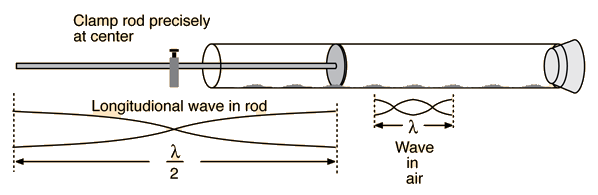# Longitudinal Waves - Kundt's Tube

Sound in air is propagated only by means of longitudinal waves: waves in which the particle's motion consists of oscillations back and forth in the direction of propagation. In a solid such as a metal rod, sound can be transmitted either by longitudinal or transverse waves. In the Kundt's tube experiment, longitudinal sound waves are produced in a metal rod, which produces them in an air column. Using the speed of sound in air and the wave relationship, the frequency of the sound and the speed of sound in the rod can be determined.The apparatus consists of a glass tube supported on a metal base as shown in the diagram. A clamp at one end of the base holds a metal rod which has a metal disk attached to one end. The rod and disk extend inside the glass tube, whose position can be adjusted to center the tube about the disk. It is important to make sure the disk is not touching the glass becuause the vibrations set up in the rod will break the glass. A stopper closes the other end of the tube. The velocity of any wave is given by:

v = fλ     (1)

where f is the frequency and l is the wavelength. When the rod is properly stroked and set into vibration, standing waves are set up in the vibrating rod. Since the rod is clamped at its center point, this point is a node (zero amplitude of motion) and the ends which are free to vibrate are antinodes (maximum amplitude of particles' motion along the direction of the rod).

When the rod is vibrating in this manner it is vibrating with its fundamental frequency and the wavelength of the standing wave is twice the length of the rod.

The vibrations of the rod are transmitted by the disk to the air in the glass tube closed at one end.The waves set up in the air in the glass tube have the same frequency as those in the rod. The waves are reflected at the closed end of the tube and the air in the tube is thus acted upon by two similar sets of waves travelling in opposite directions. When the length of the air column is some multiple of half wavelengths, the two oppositely travelling waves produce standing waves. The standing waves are characterized by alternate points of maximum and minimum disturbance called respectively nodes and antinodes. These nodes and antinodes may be detected by cork dust placed in the tube, the cork dust showing characteristic striated vibration patterns at the antinodes. The distance between two successive patterns is therefore one-half the wave length of the sound in the air.

 Kundt tube experiment
Index

Wave concepts

 HyperPhysics***** Sound R Nave
Go Back